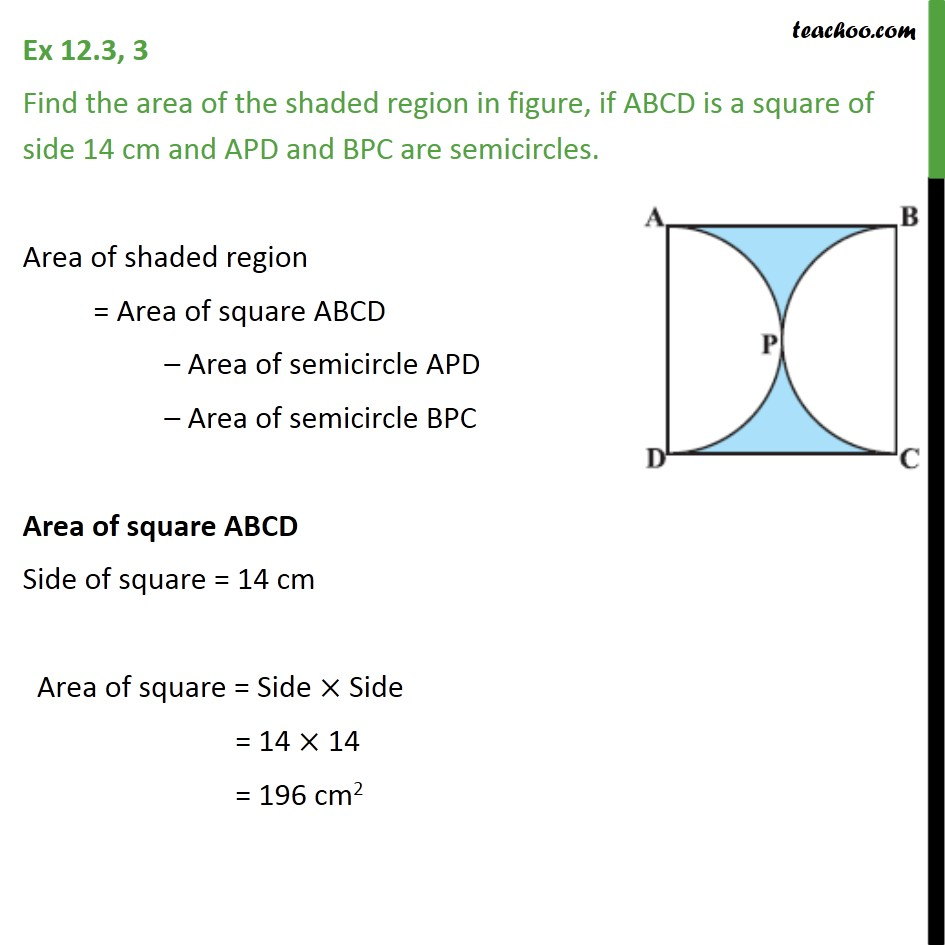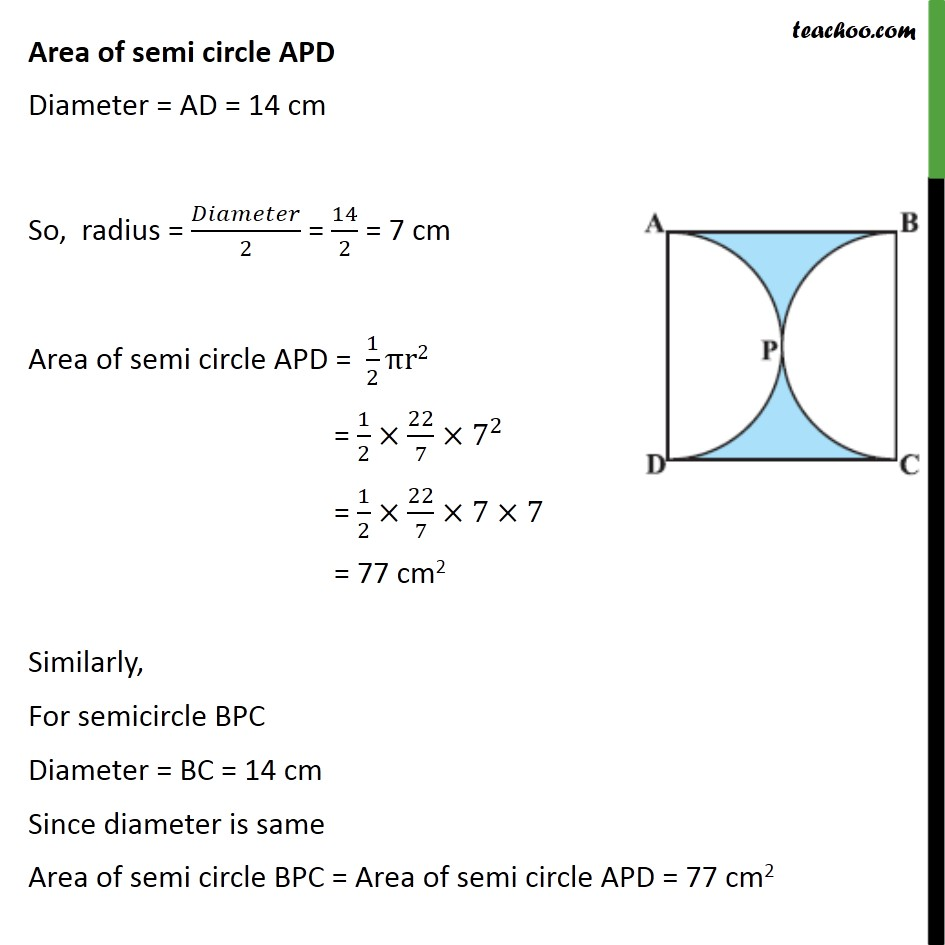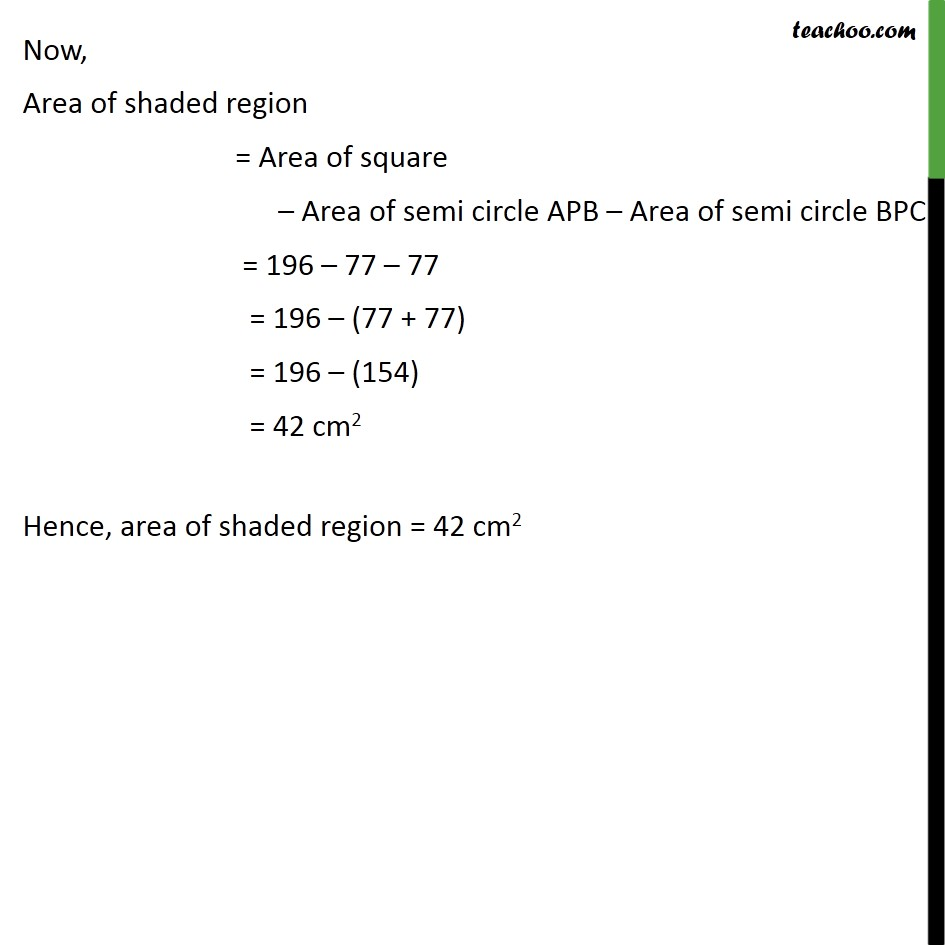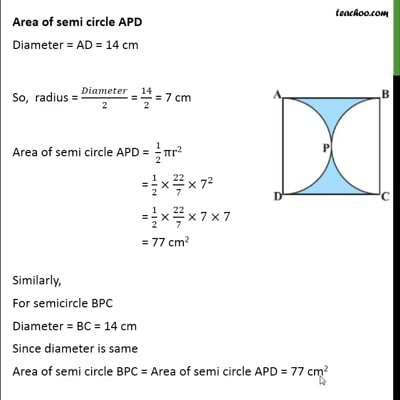Ex 12.3

Chapter 12 Class 10 Areas related to Circles (Term 1)
Serial order wiseThis video is only available for Teachoo black users

### Transcript

Ex 12.3, 3 Find the area of the shaded region in figure, if ABCD is a square of side 14 cm and APD and BPC are semicircles. Area of shaded region = Area of square ABCD Area of semicircle APD Area of semicircle BPC Area of square ABCD Side of square = 14 cm Area of square = Side Side = 14 14 = 196 cm2 Area of semi circle APD Diameter = AD = 14 cm So, radius = /2 = 14/2 = 7 cm Area of semi circle APD = 1/2 r2 = 1/2 22/7 7^2 = 1/2 22/7 7 7 = 77 cm2 Similarly, For semicircle BPC Diameter = BC = 14 cm Since diameter is same Area of semi circle BPC = Area of semi circle APD = 77 cm2 Now, Area of shaded region = Area of square Area of semi circle APB Area of semi circle BPC = 196 77 77 = 196 (77 + 77) = 196 (154) = 42 cm2 Hence, area of shaded region = 42 cm2